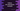# Python Set intersection example# Python Set intersection example :

Union and intersection are two important set-operations. In this blog post, we are going to discuss the intersection of two sets in Python. An intersection is used to find out the common elements between two Sets. Symbol ‘∩’ is used to denote intersection. Set contains common elements and the intersection of two sets will find out all common elements between the two sets.

For example :

In the above example, we have two Set A _and _B _and we are finding out the intersection between them _A∩B. The intersection is the common elements between A and B. From the above example, it is clear that A∩B = B∩C, i.e. the final intersection result is same irrespective of the order.

The intersection is used to find out the common elements only between two sets. But if we need to find out the intersection of more than two sets, we can perform a chain intersection. For example, if we need to find out the intersection of set A, B and C, we can first find out the intersection between A and B. Then we can find out the final intersection by calculating the intersection of C _with this _result. Using this approach, we can find the intersection of any number of Sets. But in python, we don’t need to calculate the intersection of multiple sets easily with only one function. We will explain it to you with an example below.

## Finding intersection in Python :

Now, let’s start the main part. How to find the intersection of two or more sets in python. Set operations in python are really easy to implement. Python provides us with different inbuilt methods to implement these operations easily.

Python comes with an inbuilt method_ intersection() to find out the intersection between two or more sets. In this blog post, we will show you how to use _intersection() to find out the intersection between multiple sets in python. The syntax of the intersection() method is as below :

``s.intersection(*t)``

It doesn’t require any extra module to import. The parameter is single or multiple sets that we want to find out the intersection with the caller set. You can pass multiple sets by separating them with a comma. This method returns the final intersection result. The result value is also a set.

### Example :

Let’s try to implement it with an example  :

``````firstSet = {1,2,3}
secondSet = {2,4,5}
thirdSet = {1,10,2,4}
print("firstSet : ",firstSet)
print("secondSet : ",secondSet)
print("thirdSet : ",thirdSet)
print("firstSet ∩ secondSet : ",firstSet.intersection(secondSet))
print("firstSet ∩ thirdSet : ",firstSet.intersection(thirdSet))
print("firstSet ∩ secondSet ∩ thirdSet : ",firstSet.intersection(secondSet,thirdSet))``````

As you can see, the first two times we have to find out the intersection between two sets but last time we have calculated the intersection between three sets. For the last print method, we are passing two comma separated sets. No extra module is required to import for an intersection. This is an inbuilt operation and we can use it directly with two or multiple sets.

### Conclusion :

intersection() is really a useful method to find out the intersection between sets in python. Using this method, we can easily find out the intersection result between two or multiple non-empty sets in python. You can copy and try to run the above example. Use Python-3 to run it. Try to implement/run the example and drop one comment below if you have any queries.